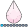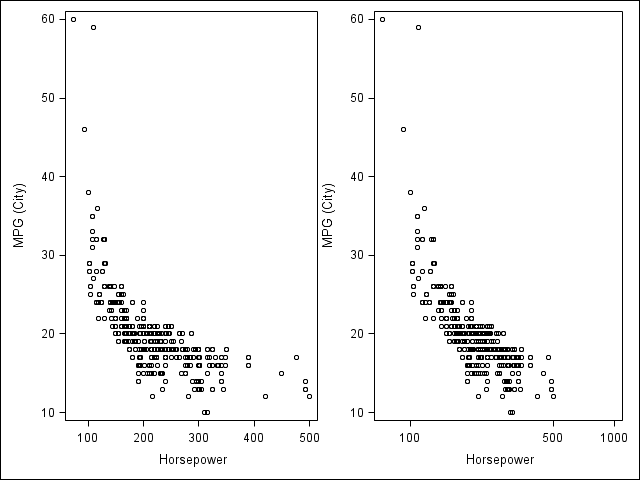## Various scales on axes proc sgpanel (SAS 9.3)

Hi,

I want to create a two-panel graph using proc sgpanel in SAS 9.3. The y-axis should be the same for the two panels, but I want one x-axis to be on a linear scale and the other on a logarithmic scale (expand). Can that be done in 9.3, or do you have any other way to create this type of graph?

Best regards,

Tommy

1 ACCEPTED SOLUTION

Accepted Solutionsdjrisks
Barite | Level 11

## Re: Various scales on axes proc sgpanel (SAS 9.3)

Hi Tommy,

You can use GTL to do this, below is an example.

proc template;
define statgraph plot_linearlog;
begingraph;
layout lattice / rows = 1 columns = 2;

layout overlay / xaxisopts=(type = linear);
scatterplot x = Horsepower y = MPG_City;
endlayout;

layout overlay / xaxisopts=(type = log logopts = (tickintervalstyle = logexpand));
scatterplot x = Horsepower y = MPG_City;
endlayout;

endlayout;
endgraph;
end;
run;

proc sgrender data = sashelp.cars template = plot_linearlog;
run;3 REPLIES 3djrisks
Barite | Level 11

## Re: Various scales on axes proc sgpanel (SAS 9.3)

Hi Tommy,

You can use GTL to do this, below is an example.

proc template;
define statgraph plot_linearlog;
begingraph;
layout lattice / rows = 1 columns = 2;

layout overlay / xaxisopts=(type = linear);
scatterplot x = Horsepower y = MPG_City;
endlayout;

layout overlay / xaxisopts=(type = log logopts = (tickintervalstyle = logexpand));
scatterplot x = Horsepower y = MPG_City;
endlayout;

endlayout;
endgraph;
end;
run;

proc sgrender data = sashelp.cars template = plot_linearlog;
run;## Re: Various scales on axes proc sgpanel (SAS 9.3)

Thanks djrisks, you opened up a new world for me when introducing GLT. A little bit tricky to sort it all out, but I could create the graph exactly as I wanted.

Tommydjrisks
Barite | Level 11

## Re: Various scales on axes proc sgpanel (SAS 9.3)

That's fine Tommy / Chris. I agree GTL is a lot more powerful and tricky, and I'm glad you were able to figure it out and create the graph exactly as you wanted.

Discussion stats
• 3 replies
• 2221 views
• 0 likes
• 2 in conversation